# Relative protein quantification for DIA-MS-based proteomics

## Introduction

The iq package, short for ion-based protein quantification, implements the MaxLFQ maximal peptide ratio extraction algorithm for data-independent acquisition (DIA) mass spectrometry (MS) based proteomics data. The algorithm was originally designed for data dependent acquisition (DDA) data (Cox et al., MCP 2014). The package also offers options for other quantitation methods including topN (using N most intense fragment ions), MeanInt (using all fragment ions), and median polish (Tukey, 1977). Finally, output from a MaxQuant experiment for DDA data can also be quantified using iq.

To install iq from CRAN (this needs to be done once)

install.packages("iq")

and the package can be loaded for usage in the usual manner

library("iq")

This vignette presents three examples for raw data processing pipelines: Spectronaut (Bruderer et al., MCP 2015), OpenSWATH (Rost et al., Nat. Biotechnol. 2014), and MaxQuant (Cox & Mann, Nat. Biotechnol. 2008). The example data are available in the project GitHub release. To keep the file size manageable, we exclude data columns not necessary for the analysis, followed by gzip compression. The user may download the data to a local working folder to run the examples.

## Spectronaut output

### An example dataset

We present an analysis of a publicly available dataset which was used in a benchmark experiment for label-free DDA and DIA proteomics (Bruderer et al., MCP 2015). The result of the MaxQuant (version 1.6.4.0) DDA search is used as a spectral library in Spectronaut version 13.0 to process DIA data. Each sample is assigned to a unique condition in Spectronaut. Subsequently, we use the conditions from C01 to C24 as sample names. We use the default Spectronaut long format export with the addition of columns: PG.Genes, PG.ProteinNames, F.ExcludedFromQuantification, F.FrgLossType, F.FrgIon, F.Charge, and F.PeakArea.

First we perform preprocessing to filter out ion fragments not used for quantification and to keep only relevant columns to keep the file size small. Note that the following code section cannot be executed here because the starting input file Bruderer15-DIA-longformat.txt is not available. To process your own data, replace Bruderer15-DIA-longformat.txt by the name of the Spectronaut export.

raw <- read.delim("Bruderer15-DIA-longformat.txt")

selected <- raw$F.ExcludedFromQuantification == "False" & raw$F.FrgLossType == "noloss" &
(is.na(raw$PG.Qvalue) | raw$PG.Qvalue <= 0.01) &
(is.na(raw$EG.Qvalue) | raw$EG.Qvalue <= 0.01)

raw <- raw[selected, c("R.Condition","PG.ProteinGroups", "EG.ModifiedSequence", "FG.Charge",
"F.FrgIon", "F.Charge", "F.PeakArea", "PG.Genes", "PG.ProteinNames")]

write.table(raw, "Bruderer15-DIA-longformat-compact.txt", sep = "\t", row.names = FALSE)

Note that if the quantification filtering option is selected when exporting the report from Spectronaut, the software already filters out entries with high protein q-values and peptide q-values. We can check that by

all(raw$PG.Qvalue <= 0.01) all(raw$EG.Qvalue <= 0.01)

We write the content of raw to a text file and gzip it to keep the file size manageable. In the following, we will continue with the compressed file Bruderer15-DIA-longformat-compact.txt.gz. Note that the user does not need to write the data to disk and read it back like in this example when processing their own data.

raw <- read.delim(gzfile("Bruderer15-DIA-longformat-compact.txt.gz"))

The MaxLFQ quantification is performed in three steps

norm_data <- iq::preprocess(raw)
protein_list <- iq::create_protein_list(norm_data)
result <- iq::create_protein_table(protein_list)

The first step iq::preprocess prepares a long-format input including removing low-intensity ions and performing median normalization. To select a threshold for removing low-intensity ions, plot a histogram of the data as follows

hist(log2(raw[, "F.PeakArea"]), 100, main = "Histogram of log2 intensities",
col = "steelblue", border = "steelblue", freq = FALSE)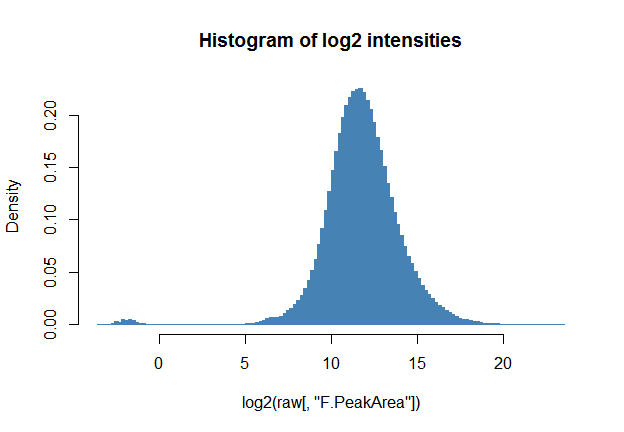Here the default threshold value of zero for log2_intensity_cutoff is appropriate as it removes the strange distribution of low-intensity data points on the left of the figure.

The default value for sample_id is "R.Condition". However, in practice we find it convenient to use "R.FileName" because one does not need to prepare the condition column in Spectronaut. The secondary_id parameter enforces the uniqueness of the peptide species for the MaxLFQ algorithm. For example, when multiple spectral libraries are used, it is possible that a fragment comes from two or more libraries. In that case, we can use secondary_id = c("EG.Library", "FG.Id", "FG.Charge", "F.FrgIon", "F.Charge"). Note that one needs to export the necessary columns from Spectronaut.

The second step iq::create_protein_list converts the long-format table to a list where each element contains the underlying data of each protein. The column names are sample names and row names fragment ions. The result can be used to visualized protein quantification for manual validation as in the next section.

The last statement iq::create_protein_table quantifies the protein list one by one.

The following statement produces a quantified protein table in a tab-separated text file output-maxLFQ.txt containing the protein identifiers in the first column, the quantified proteins, and the annotation text in the last column. This file can be opened by a spreadsheet application such as Excel.

write.table(cbind(Protein = rownames(result$estimate), result$estimate,
annotation = result$annotation), "output-maxLFQ.txt", sep = "\t", row.names = FALSE) ### Protein visualization The function iq::plot_protein() plots the underlying data for individual proteins. By default, the names of fragment ions are displayed on the right panel, which can be disabled by setting parameter split to NULL. iq::plot_protein(protein_list$P00366, main = "Protein P00366", split = NULL)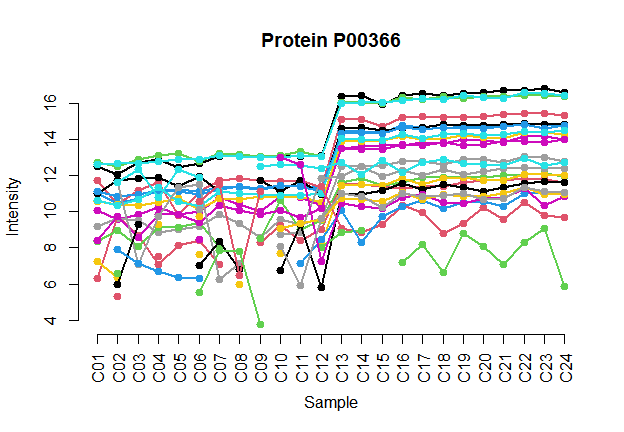By attaching the result of the quantitation algorithm to the data table, we can demonstrate both protein quantitative values and the underlying data. Here we set the colors of fragment ions to gray.

iq::plot_protein(rbind(protein_list$P00366, MaxLFQ = iq::maxLFQ(protein_list$P00366)$estimate), main = "MaxLFQ quantification of P00366", col = c(rep("gray", nrow(protein_list$P00366)), "green"),
split = NULL)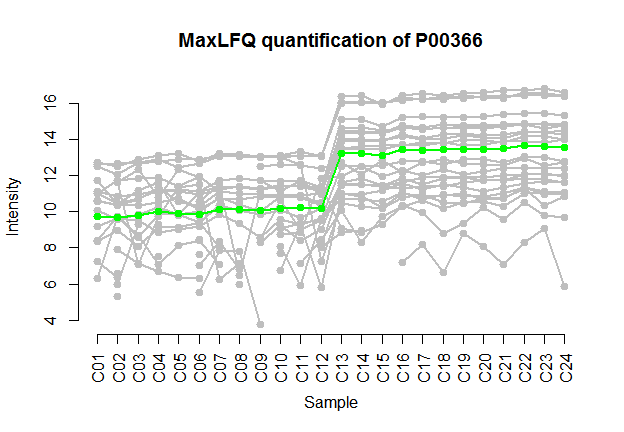The names of constituent ions are displayed on the right when split is not NULL. We can pass additional graphical parameters to fine-tune the plot. For example, in the following we make the font size smaller by setting cex to 0.4 due to the restricted plotting space.

iq::plot_protein(protein_list$P00366, main = "Protein P00366", cex = 0.4)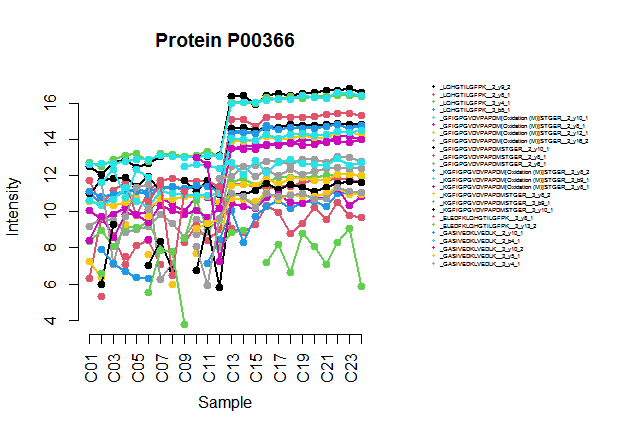We can also use the protein plotting function to display different quantitative methods for evaluation. For example, here we show the quantitative values of a spike-in protein using MaxFLQ and the spike-in ground truth values (rescaled so that the means of the two are the same). MaxLFQ_estimate <- iq::maxLFQ(protein_list$P12799)$estimate ground_truth <- log2(rep(c(200, 125.99, 79.37, 50, 4, 2.52, 1.59, 1), each = 3)) ground_truth <- ground_truth - mean(ground_truth) + mean(MaxLFQ_estimate) iq::plot_protein(rbind(MaxLFQ = MaxLFQ_estimate, Groundtruth = ground_truth), main = "P12799 - MaxLFQ versus groundtruth", split = 0.75, col = c("green", "gold"))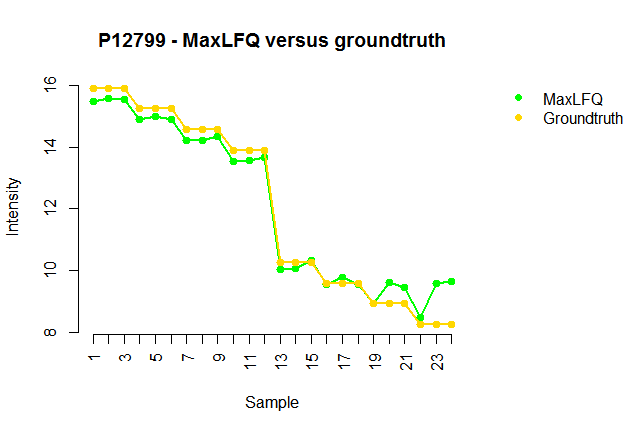### Extracting additional protein annotation The input data might contain extra annotations for the proteins. We provide a convenient function to extract additional annotation. The following script produces the same quantitative values as before, but with additional columns for gene names and protein names. extra_names <- iq::extract_annotation(rownames(result$estimate),
raw,
annotation_columns = c("PG.Genes", "PG.ProteinNames"))

write.table(cbind(Protein = rownames(result$estimate), extra_names[, c("PG.Genes", "PG.ProteinNames")], result$estimate,
annotation = resultannotation), "output-maxLFQ-annotation.txt", sep = "\t", row.names = FALSE) ## OpenSWATH output We download OpenSWATH data from the publication of Schubert et al. (Cell Host & Microbe 2015). The data is in a long format with fragment ions and their corresponding intensities are concatenated in two entries for each peptide. We separate these entries into an extended long format. Again, we will only keep relevant columns and compress the result. Thus, the user can skip this code section and continue to the next section using the data available in the project GitHub release. tab <- read.delim("./Mtb_feature_alignment_requant_filtered_max10_fixed_noUPS.tsv", stringsAsFactors = FALSE) tabCondition[tab$Condition == "d20_6h"] <- "d20_06h" tab_list <- vector("list", nrow(tab)) for (i in 1:nrow(tab)) { a <- unlist(strsplit(tab[i, "aggr_Fragment_Annotation"], ";")) b <- unlist(strsplit(tab[i, "aggr_Peak_Area"], ";")) tab_list[[i]] <- NULL for (j in 1:length(a)) { tab[i, "aggr_Fragment_Annotation"] <- a[j] tab[i, "aggr_Peak_Area"] <- b[j] tab_list[[i]] <- rbind(tab_list[[i]], tab[i,]) } } tab_extended <- do.call(rbind.data.frame, tab_list) quant <- as.double(tab_extended$aggr_Peak_Area)

short_name <- paste(tab_extended$Condition, tab_extended$BioReplicate,
tab_extended$Run, sep = "_") tab_small <- cbind(tab_extended[, c("ProteinName", "FullPeptideName", "Charge", "aggr_Fragment_Annotation")], quant, short_name) write.table(tab_small, "Schubert15-OpenSWATH.txt", sep = "\t", row.names = FALSE) ### Protein quantification First we load the compressed data prepared by the previous step tab_small <- read.delim(gzfile("Schubert15-OpenSWATH.txt.gz")) and check the head of the data file head(tab_small) #> ProteinName FullPeptideName Charge aggr_Fragment_Annotation quant #> 1 1/Rv0244c_fadE5 ALGHGEFSDVDVDTAR 2 y9_1 1200.270 #> 2 1/Rv0244c_fadE5 ALGHGEFSDVDVDTAR 2 y7_1 1635.659 #> 3 1/Rv0244c_fadE5 ALGHGEFSDVDVDTAR 2 y5_1 2130.497 #> 4 1/Rv0244c_fadE5 ALGHGEFSDVDVDTAR 2 y12_1 2324.244 #> 5 1/Rv0244c_fadE5 ALGHGEFSDVDVDTAR 2 y10_1 2853.498 #> 6 1/Rv0244c_fadE5 ALGHGEFSDVDVDTAR 2 y9_1 27360.707 #> short_name #> 1 d00_1_1 #> 2 d00_1_1 #> 3 d00_1_1 #> 4 d00_1_1 #> 5 d00_1_1 #> 6 d05_1_2 Then we plot a histogram of the data to examine the data distribution hist(log2(tab_small[, "quant"]), 100, main = "Histogram of log2 intensities", col = "steelblue", border = "steelblue", freq = FALSE)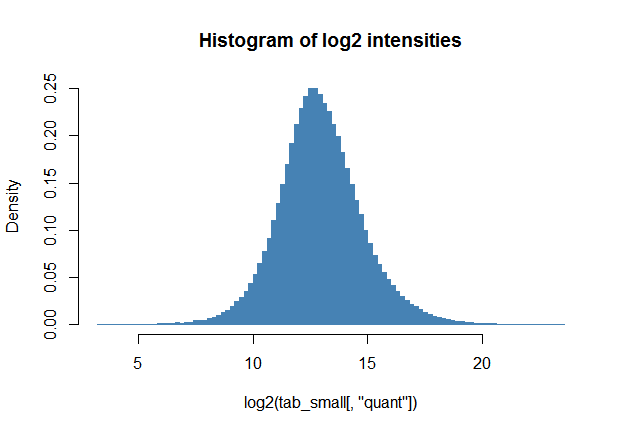Here we do not observe abnormal low-intensity values, and the default value intensity cutoff of zero is good. We follow the same procedure as for Spectronaut output for protein quantification. Nevertheless, we have to adapt the column names appropriately. Unique values in the primary_id column form the list of proteins to be quantified. A concatenation of the columns in secondary_id determines the fragment ions used for quantification. Unique values in the sample_id column form the list of samples. Finally, column intensity_col specifies the quantitative values. norm_data <- iq::preprocess(tab_small, primary_id = "ProteinName", secondary_id = c("FullPeptideName", "Charge", "aggr_Fragment_Annotation"), sample_id = "short_name", intensity_col = "quant") protein_list <- iq::create_protein_list(norm_data) result <- iq::create_protein_table(protein_list) write.table(cbind(Protein = rownames(result$estimate),
result$estimate, annotation = result$annotation),
"Schubert-output-maxLFQ.txt", sep = "\t", row.names = FALSE)

The resulting output text file Schubert-output-maxLFQ.txt can be loaded into a spreadsheet application.

## MaxQuant output

The MaxLFQ algorithm is implemented in the software package MaxQuant. Nevertheless, there are cases in which MaxQuant fails in the label-free quantification step. One possible solution is to run MaxQuant without normalization and derive the MaxLFQ values using iq.

### Read the protein group data

First, we read the protein quantification from MaxQuant output, ignoring entries detected in the reversed fasta database. The protein group table provides links to the underlying data in the MaxQuant evidence.txt file. When LFQ values are available, we can store them in a table to compare the result of the iq implementation to that of MaxQuant.

dda <- read.delim(gzfile("proteinGroups.txt.gz"))
dda <- subset(dda, Reverse == "")    # remove reversed entries
rownames(dda) <- dda[,"Protein.IDs"] # use protein group ids as rownames
lfq <- grep("^LFQ", colnames(dda))
dda_log2 <- log2(dda[, lfq])
dda_log2[dda_log2 == -Inf] <- NA

colnames(dda_log2) <- sprintf("C%02d", 1:24)

### Building up a protein list from the MaxQuant evidence file

We first perform median normalization on intensities in the evidence.txt file. Subsequently, we produce a list of protein as in the case of Spectronaut output in a variable p_list.

evidence <- read.delim(gzfile("evidence.txt.gz"), stringsAsFactors = FALSE)

rownames(evidence) <- evidence[, "id"]

# median normalization
ex <- paste0(paste0("sample", rep(1:8, each=3),"_R0"), rep(1:3, 8))
ex_median <- rep(NA, length(ex))
names(ex_median) <- ex
for (i in ex) {
tmp <- subset(evidence, Experiment == i)
ex_median[i] <- median(tmp[,"Intensity"], na.rm = TRUE)
}
f <- mean(ex_median)/ex_median
evidence[, "Intensity"] <- evidence[, "Intensity"] * f[evidence[, "Experiment"]]

# create a protein list
p_list <- list()

for (i in 1:nrow(dda)) {

tmp <- unlist(strsplit(as.character(dda[i, "Evidence.IDs"]), ";"))
a <- evidence[tmp,]
b <- data.frame(cn = a[, "Experiment"],
rn = paste(a[, "Modified.sequence"], a[, "Charge"], sep="_"),
quant = a[, "Intensity"])
b <- b[!is.na(b$quant),] m <- matrix(NA, nrow = length(unique(b$rn)), ncol = length(ex),
dimnames = list(unique(b$rn), ex)) if (nrow(b) > 0) { for (j in 1:nrow(b)) { rn <- as.character(b$rn[j])
cn <- as.character(b$cn[j]) if (is.na(m[rn, cn])) { m[rn, cn] <- b$quant[j]
} else {
m[rn, cn] <- m[rn, cn] + b$quant[j] } } p_list[[rownames(dda)[i]]] <- log2(m) } else { p_list[[rownames(dda)[i]]] <- NA } } Once the protein list is created, we can perform the MaxLFQ algorithm for DDA as for DIA data. An example for MaxLFQ by MaxQuant and iq is shown here (rescaled so that the means of the two quantification values are equal). w1 <- iq::maxLFQ(p_list$A1L0T0)$estimate w2 <- as.numeric(dda_log2["A1L0T0", ]) w2 <- w2 - mean(w2, na.rm = TRUE) + mean(w1, na.rm = TRUE) tmp <- rbind(p_list$A1L0T0,
MaxLFQ by iq = w1,
MaxLFQ by MaxQuant = w2)
colnames(tmp) <- sprintf("C%02d", 1:24)

iq::plot_protein(tmp,
main = "A1L0T0", cex = 0.5, split = 0.65,
col = c(rep("gray", nrow(p_list$A1L0T0)), "green", "blue"))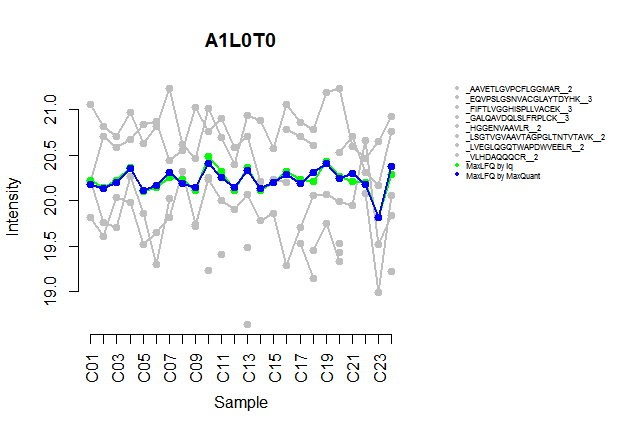Finally, we write out the result of MaxLFQ quantification to a text file. output_mq <- iq::create_protein_table(p_list) write.table(cbind(Protein = rownames(output_mq$estimate),
output_mq$estimate, annotation = output_mq$annotation),
"output_IQ_LFQ.txt", sep = "\t", row.names = FALSE)

The tab-separated text file output_IQ_LFQ.txt now contains the MaxLFQ values by the package iq implementation.

## Other quantitation methods: topN, MeanInt, and median polish

We also implement the topN method, the MeanInt method, and the median polish method by introducing the method parameter to the iq::create_protein_table() function. We create outputs for all different methods in the following. Here, the variable protein_list is from the Spectronaut or OpenSWATH example.

# default MaxLFQ
output <- iq::create_protein_table(protein_list)

# median polish
output <- iq::create_protein_table(protein_list, method = "median_polish")

# top 3
output <- iq::create_protein_table(protein_list, method = "topN", N = 3)

# top 5
output <- iq::create_protein_table(protein_list, method = "topN", N = 5)

# MeanInt in the original intensity space
output <- iq::create_protein_table(protein_list, method = "meanInt",
aggregation_in_log_space = FALSE)

For the topN and MeanInt method, the aggregating operation can be carried out in the log space by default or in the original intensity space by setting the parameter aggregation_in_log_space to FALSE.